# NCERT Solutions for Class 6 Maths Chapter 4 Basic Geometrical Ideas

In this page, you will get Chapter 4 Basic Geometrical Ideas NCERT Solutions for Class 6 Maths that will useful in grasping the important concepts of the chapter properly. You can also Download PDF of Class 6 Maths Chapter 4 Basic Geometrical Ideas NCERT Solutions which will improve the learning behaviour of the students. A students can obtain maximum marks in the exams by practising these NCERT Solutions.

Chapter 4 NCERT Solutions will give good experience and provide opportunities to learn new things. These NCERT Solutions are helpful resources that can help you not only cover the entire syllabus but also provide in depth analysis of the topics. It will help you in analyzing the problems and answering it with precision and the right concepts.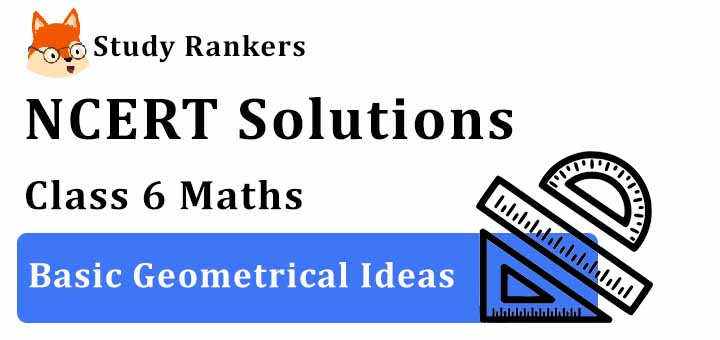Page No: 74

Exercise 4.1

1. Use the figure to name:
(a) Five points
(b) A line
(c) Four rays
(d) Five line segments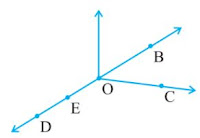(a) In the given figure five points are B,O,E,D,C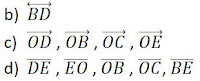2. Name the line given in all possible (twelve) ways, choosing only two letters at a time from the four given.Page No: 75

3. Use the figure to name:
(a) Line containing point E.
(b) Line passing through A.
(c) Line on which O lies
(d) Two pairs of intersecting lines.4. How many lines can pass through (a) one given point? (b) two given points?

(a) From a single point infinite line can pass.
(b) From two given points one line can pass through.

5. Draw a rough figure and label suitably in each of the following cases: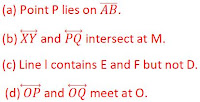(a) True
(b) True
(c) True
(d) False
(e) False
(f) False
(g) True
(h) False
(i) False
(j) False
(k) True

Page No. 80

Exercise 4.2

1. Classify the following curves as (i) Open or (ii) Closed.(a) Open
(b) Closed
(c) Open
(d) Closed
(e) Closed

2. Draw rough diagrams to illustrate the following:
(a) Open curve
(b) Closed curve.3. Draw any polygon and shade its interior.4. Consider the given figure and answer the questions:
(a) Is it a curve?
(b) Is it closed?(a) True
(b) True

5. Illustrate, if possible, each one of the following with a rough diagram:
(a) A closed curve that is not a polygon.
(b) An open curve made up entirely of line segments.
(c) A polygon with two sides.(c) This is not possible as the polygon having the least number of sides is a triangle, which has three sides in it.

Page No. 80

Exercise 4.3

1. Name the angles in the given figure.2. In the given diagram, name the point(s)(a) In the interior of ∠DOE
(b) In the exterior of ∠EOF
(c) On ∠EOF

(a) A
(b) C,A,D
(c) B,E,O,F

3. Draw rough diagrams of two angles such that they have
(a) One point in common.
(b) Two points in common.
(c) Three points in common.
(d) Four points in common.
(e) One ray in common.

(a) ∠COD and ∠AOB have point O in common.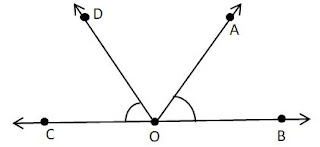(b) ∠AOB and ∠BOC have points O and B in common.(c) ∠AOB and ∠BOC have points O, E, B in common.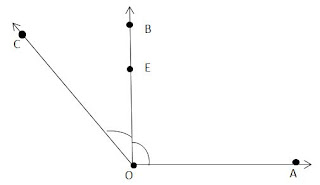(d) ∠BOA and ∠COA have points O, E, D, A in common.(e) Ray OC is common between ∠BOC and ∠AOC.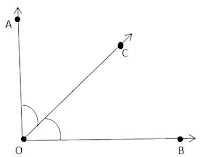Page No. 81

Exercise 4.4

1. Draw a rough sketch of a triangle ABC. Mark a point P in its interior and a point Q in its exterior. Is the point A in its exterior or in its interior?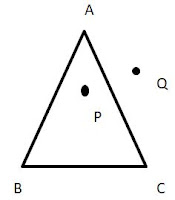2. (a) Identify three triangles in the figure.
(b) Write the names of seven angles.
(c) Write the names of six line segments.
(d) Which two triangles have ∠B as common?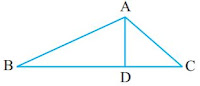(c) AB, BC, CA, AD, BD, CD
(d) ΔABD and ΔABC

Page No. 82

Exercise 4.5

1. Draw a rough sketch of a quadrilateral PQRS. Draw its diagonals. Name them. Is the meeting point of the diagonals in the interior or exterior of the quadrilateral?Diagonal PR and diagonal SQ meet at O, which is inside the quadrilateral.

2. Draw a rough sketch of a quadrilateral KLMN. State,
(a) two pairs of opposite sides,
(b) two pairs of opposite angles,
(c) two pairs of adjacent sides,
(d) two pairs of adjacent angles.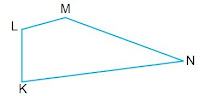(a)Two pairs of opposite sides.
(b)Two pairs of opposite angles.

3. Investigate :
Use strips and fasteners to make a triangle and a quadrilateral.
Try to push inward at any one vertex of the triangle. Do the same to the quadrilateral.
Is the triangle distorted? Is the quadrilateral distorted? Is the triangle rigid? Why is it that structures like electric towers make use of triangular shapes and not quadrilaterals?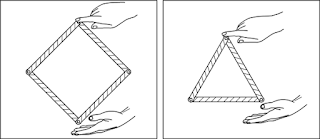No, the triangle is not distorted but the quadrilateral is distorted.
Yes, the triangle is rigid.

Structures like electric towers make use of triangular shape as the structure of triangles are rigid and hence cannot be distorted.

Page No. 84

Exercise 4.6

1. From the figure, identify :
(a) the centre of circle
(c) a diameter
(d) a chord
(e) two points in the interior
(f) a point in the exterior
(g) a sector
(h) a segment(a) O is the centre.
(b) Three radii are OA, OB and OC
(c) A diameter: AC
(d) A chord: ED
(e) Interior points: O, P
(f) Exterior point: Q
(g) A sector: OAB
(h) A segment: ED

2. (a) Is every diameter of a circle also a chord?
(b) Is every chord of a circle also a diameter?

(a) Yes, every diameter of a circle is also a chord.

(b) No, every chord of a circle is not a diameter.

3. Draw any circle and mark
(a) its centre
(c) a diameter
(d) a sector
(e) a segment
(f) a point in its interior
(g) a point in its exterior
(h) an arc(a) Centre: O
(c) A diameter: AC
(d) A sector: OAB
(e) A segment: ED
(f) A point in its interior: P
(g) A point in its exterior: Q
(h) An arc: DC

4. Say true or false: (a) Two diameters of a circle will necessarily intersect.
(b) The centre of a circle is always in its interior.

(a) True

(b) True

## NCERT Solutions for Class 6 Maths Chapter 4 Basic Geometrical Ideas

Class 6 NCERT Solutions are prepared as per the accordance of latest CBSE guidelines so you can score maximum marks. The term 'geometry' is derived from the Greek word 'geometron'. We will see various terms such as points, line segment, ray, intersecting lines etc.

• In geometry, dots are used to represent points. A point is used to represent any specific location or position. It neither has any size, nor dimensions such as length or breadth.

• A line segment is defined as the shortest distance between two points.

• A line is made up of an infinite number of points that extend indefinitely in either direction.

You can find exercisewise NCERT Solutions from the links given below which will improve studying habits and set a good foundation for your future goals. It will make you understand the topics in most simple manner and grasp it easily to perform better.

• Exercise 4.1 Chapter 4 Class 6 Maths NCERT Solutions
• Exercise 4.2 Chapter 4 Class 6 Maths NCERT Solutions
• Exercise 4.3 Chapter 4 Class 6 Maths NCERT Solutions
• Exercise 4.4 Chapter 4 Class 6 Maths NCERT Solutions
• Exercise 4.5 Chapter 4 Class 6 Maths NCERT Solutions
• Exercise 4.6 Chapter 4 Class 6 Maths NCERT Solutions

These NCERT Solutions for Class 6 are prepared Studyrankers subject matter experts will make you well versed in variety of topics and you will be able to instantly recollect your ideas and shape your answers. These help you in getting a deeper understanding of various topics.

### NCERT Solutions for Class 6 Maths Chapters:

 Chapter 1 Knowing Our Numbers Chapter 2 Whole Numbers Chapter 3 Playing with Numbers Chapter 5 Understanding Elementary Shapes Chapter 6 Integers Chapter 7 Fractions Chapter 8 Decimals Chapter 9 Data Handling Chapter 10 Mensuration Chapter 11 Algebra Chapter 12 Ratio and Proportion Chapter 13 Symmetry Chapter 14 Practical Geometry

FAQ on Chapter 4 Basic Geometrical Ideas

#### How many exercises in Chapter 4 Basic Geometrical Ideas?

There are total 6 exercises in the Chapter 4 Class 6 Maths textbook which will allow the students to evaluate their learning almost immediately and getting command over the chapter. These NCERT Solutions will improve the understanding of the chapter and guide student in a better way.

#### What do you mean by Intersecting lines?

If two lines pass through a point, then we say that the two lines intersect at that point. Thus, if two lines have one point in common, then they are called intersecting lines.

#### What do you mean by Curves?

Curves can be defined as figures that flow smoothly without a break. A line is also a curve, and is called a straight curve. Curves that do not intersect themselves are called simple curves. The end points join to enclose an area. Such curves are called closed curves.

#### What do you mean by Parallel lines?

In a plane, if two lines have no point in common, then they are said to be parallel or non- intersecting lines. Parallel lines never meet, cut or cross each other.